# The Sine Rule

The sine rule is a property that is true for all triangles drawn on a flat surface. It can be verified by drawing a triangle on a piece of paper and measuring all three sides and all three angles.

When we draw a triangle, we label the vertices with upper case letters. The side opposite each vertex takes the corresponding lowercase letter.The sine rule states thatIn other words, dividing a side length by the sine of its opposite angle produces a constant. The constant belongs to the triangle. It isn’t a universal constant like the number. We getwhen we divide the circumference of any circle by its diameter.

Notice the constant for all three calculations for the triangle drawn below. Now move the points around to see that the constant depends on the triangle.

For a right angled triangle the constant is just the hypotenuse. Move the points in the applet above so that one of the angles is 90 degrees or as close as possible. Note the value of the constant on the right is equal to the length of the hypotenuse.

We can make sense of this using SOH CAH TOA as follows:For example, side lengththerefore,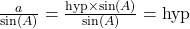When the triangle is not right angled, what does the valuerepresent? This is a really neat question for another page.

SOH CAH TOA is limited to right angle triangles. The sine rule is true for all triangles,  right angled or not.

In order to apply the sine rule we must know one opposite side/angle pair. Here are some examples.

# Example 1: Solving a triangle by finding a side first

To solve a triangle means to calculate all missing sides and angles.We can use the sine rule to solve this triangle because the angleis opposite the side.

From the sine rule we know that because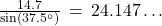then. This allows us to figure out the value.

However, we can skip this line of work and go straight to this statement:Multiplying both sides by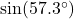we have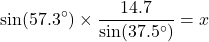ThereforeWe can calculate the angle markedbecause the angles in a triangle add to 180 degrees.It might be tempting to use the Pythagorean Theorem to calculate the side markedhowever we can only use this if the triangle is right angled, which ours is not. So we will use the sine rule a second time: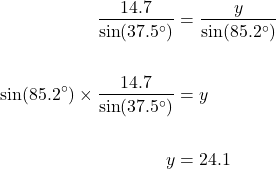Now our triangle is solved.

# Example 2: Solving a triangle by finding an angle first

The sine rule can be written in two forms: either asor asWhen finding an angle, it is easier to use the second form although either will work.The given opposite pair is the side with lengthand the angleThereforeAgain we can calculate the angle markedbecause the angles in a triangle add to 180 degrees.Lastly we use the sine law again to solve for the remaining side:Now our example 2 is solved.

# Example 3: No side-angle pair?

Solve to find the angle marked.In this example, we see don’t have a side-angle pair given. Sometimes there is no side-angle pair and we can’t use the sine rule – we have to use the cosine rule.

However in this case you may notice that we have two angles, and so the third is a simple calculation:Now we have a side/angle pair that we can use to calculate:The final side calculates to 11 units correct to the nearest unit.

# Example 4: The angle is obtuse

Recall that the answer to a sine equation such ashas two solutions betweenand– one is acute, the other is obtuse. (see Trig Equations)

To calculate the acute solution, we simply useon the calculator.To calculate the obtuse solution, we need to subtract from 180 degrees:When using the sine rule to calculate an angle it is important to know whether the angle required is acute or obtuse.

In this question we are required to find an angle that is obtuse:We set up the sine law as before to find an angle (example 2):So far so good. This is the point where we need to decide to finish this differently:

Because the angle we require is obtuse (we are told so in the words of the question or we know so for some other reason), we calculateby subtracting from: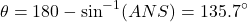# Practice:

You can practice using the sine rule online at transum math

or

# Proof

The sine law can be explained in a number of ways. This way only requires familiarity with SOH CAH TOA,